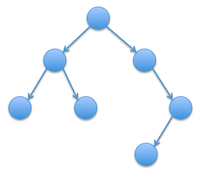## Part 1: Measuring Inefficiency (30%)

In class, we analyzed the `add` method for the BinaryTree class. Because each recursive call involves counting the number of nodes in each subtree (an O(N) operation) and the number of calls depends upon the height of the tree (which is O(log N) in a balanced tree), adding an item using this method will be O(N log N). Design a program that will verify this analysis experimentally using techniques similar to HW3. Provide statistics from executions of your program and a coherent argument as to why the statistics support the claim that `add` is O(N log N).

## Part 2: Inductive Proofs (30%)

Provide an inductive proof of each of the following statements about binary trees.
1. In any non-empty, full binary tree, there are more nodes on the bottom (deepest) level than all other levels combined.
2. In any non-empty binary tree, there will always be more empty children (i.e., null left or right fields within nodes) than children (i.e., non-null fields)

## Part 3: Binary Tree Methods (40%)

The height of a tree is defined to be the length of the longest path (in terms of number of nodes) from the root to a leaf. The weight of a tree is defined to be the sum of all the node depths. For example, the height of the tree shown below is 4 while its weight is 1 + 2 + 2 + 3 + 3 + 3 + 4 = 18.Both of these properties can also be defined recursively. The height of a nonempty binary tree is the maximum of the heights of the two subtrees plus 1. The weight of a nonempty binary tree is the sum of the weights of the two subtrees plus the number of nodes in the tree.

Add methods to the BinaryTree class named `height` and `weight`, that calculate and return these tree properties. Modify the main method of your `BinaryTree` class so that it tests each of these methods. Part of your grade will depend upon the thoroughness of your tests. Also, don't forget to add your name to the @author comment!